The OpenFOAM Foundation
sensibleEnthalpy< Thermo > Class Template Reference

Thermodynamics mapping class to expose the sensible enthalpy functions. More...

## Public Member Functions

sensibleEnthalpy ()
Construct. More...

scalar Cpv (const Thermo &thermo, const scalar p, const scalar T) const

scalar CpByCpv (const Thermo &thermo, const scalar p, const scalar T) const
Cp/Cp []. More...

scalar HE (const Thermo &thermo, const scalar p, const scalar T) const

scalar THE (const Thermo &thermo, const scalar h, const scalar p, const scalar T0) const
Temperature from sensible enthalpy. More...

## Static Public Member Functions

static word typeName ()
Return the instantiated type name. More...

static word energyName ()

## Detailed Description

### template<class Thermo> class Foam::sensibleEnthalpy< Thermo >

Thermodynamics mapping class to expose the sensible enthalpy functions.

Definition at line 44 of file sensibleEnthalpy.H.

## ◆ sensibleEnthalpy()

 sensibleEnthalpy ( )
inline

Construct.

Definition at line 52 of file sensibleEnthalpy.H.

## ◆ typeName()

 static word typeName ( )
inlinestatic

Return the instantiated type name.

Definition at line 59 of file sensibleEnthalpy.H.

## ◆ energyName()

 static word energyName ( )
inlinestatic

Definition at line 66 of file sensibleEnthalpy.H.

References sensibleEnthalpy< Thermo >::Cpv().

Here is the call graph for this function: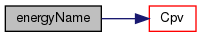## ◆ Cpv()

 scalar Cpv ( const Thermo & thermo, const scalar p, const scalar T ) const
inline

Definition at line 73 of file sensibleEnthalpy.H.

References sensibleEnthalpy< Thermo >::CpByCpv().

Referenced by sensibleEnthalpy< Thermo >::energyName().

Here is the call graph for this function: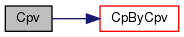Here is the caller graph for this function: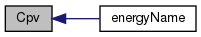## ◆ CpByCpv()

 scalar CpByCpv ( const Thermo & thermo, const scalar p, const scalar T ) const
inline

Cp/Cp [].

Definition at line 84 of file sensibleEnthalpy.H.

References sensibleEnthalpy< Thermo >::HE().

Referenced by sensibleEnthalpy< Thermo >::Cpv().

Here is the call graph for this function: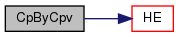Here is the caller graph for this function: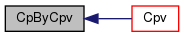## ◆ HE()

 scalar HE ( const Thermo & thermo, const scalar p, const scalar T ) const
inline

Definition at line 95 of file sensibleEnthalpy.H.

References sensibleEnthalpy< Thermo >::THE().

Referenced by sensibleEnthalpy< Thermo >::CpByCpv().

Here is the call graph for this function: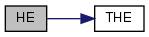Here is the caller graph for this function: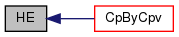## ◆ THE()

 scalar THE ( const Thermo & thermo, const scalar h, const scalar p, const scalar T0 ) const
inline

Temperature from sensible enthalpy.

given an initial temperature T0

Definition at line 107 of file sensibleEnthalpy.H.

Referenced by sensibleEnthalpy< Thermo >::HE().

Here is the caller graph for this function: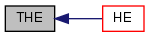The documentation for this class was generated from the following file: